## [要闻] 科学前沿要闻-009 2016-3-26

Keep-Left Behavior Induced by Asymmetrically Profiled Walls

C. L. N. Oliveira,1, A. P. Vieira ,D. Helbing, J. S. Andrade,

Jr .H. J. Herrmann

(PHYSICAL REVIEW X 6, 011003 (2016))

In the present work, we show that corridors with asymmetrically profiled walls can induce organization in the flow of pedestrians. Precisely, the key element of our approach is to use walls having the geometry of a ratchet, in the present work, we show that corridors with asymmetrically profiled walls can induce organization in the flow of pedestrians. Precisely, the key element of our approach is to use walls having the geometry of a ratchet, i.e., a zigzag structure such as the one shown in Fig. 1(a).We consider that all pedestrians (modeled here as self-driven particles) in the channel are identical except that half of them are downward movers, i.e., driven by a force downward, while the other half are upward movers, i.e., driven by a force upward. These forces try to impose a constant drift velocity. A repulsive potential acts between particles and with the walls, while a stochastic force introduces disorder. The flow orientation along the walls strongly depends on the system’s parameters. Moreover, as we will see later, increasing the strength of the disorder may suppress organization.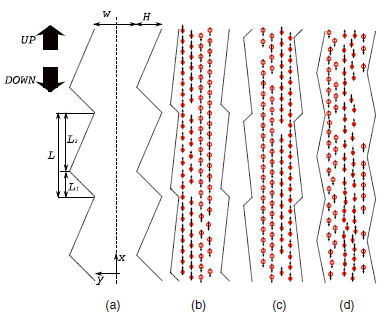FIG. 1. Channel geometry and final configurations of pedestrians for different asymmetry parameters. (a) Channel, of depth H and width W, through which a preferred flow orientation of particles is induced. The meaning of geometric parameters is indicated. (b–d) Typical configurations in the steady state, where the particles are keeping left in asymmetric wall channels but are mixed in symmetric ones. Upward movers (downward movers) are represented by full (hollow) particles. The velocity of each particle is indicated by arrows.

In Fig. 2, we show, for different drift velocities in systems without noise, how the degree of asymmetry b changes the lanes and induces preferential sides of the particle flows in the channel. Interestingly, the larger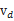, the more abrupt the change between the ordered states,, as b changes. For large positive values of b, we find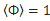; i.e., everybody is keeping left perfectly. For large negative values of b, we find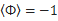, corresponding to everybody keeping right. Finally, there is a mixed state for b close to zero, where no preferred side is observed.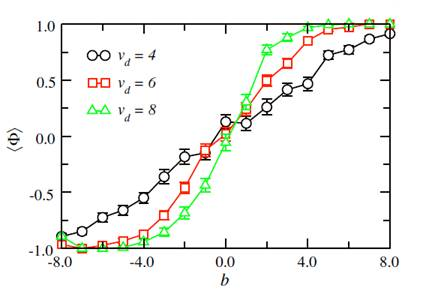FIG. 2. Average order parameter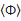as a function of the asymmetry parameter b, for three different values of the drift velocity. We see that order is only induced for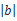> 0. The slower, the highershould be in order to achieve a two-lane pattern. The remaining parameters, including the lack of noise, are the same as in Fig. 1.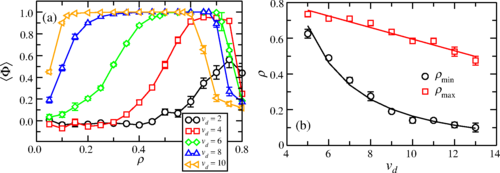FIG.3 Dependence of density on drift velocity for ordered states. In panel (a), the average order parameter Φ is shown as a function of the density for θ=0b=8H=2, and several values of the drift velocity vd. In panel (b), we show the maximum (red squares) and minimum (black circles) densities for which Φ=1, as functions of the drift velocity. A power-law fit for the minimum density yields ρmin=17.6v−2.03d, whereas a linear fit for the maximum density gives ρmax=0.919−0.032vd. The equations extracted from the fits meet at two points, namely, vd≈4.67 and vd≈28.09.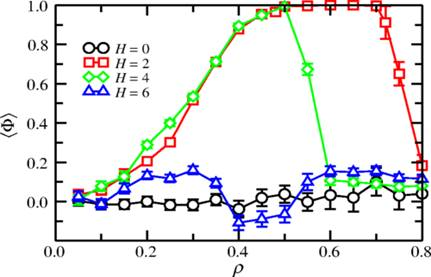FIG. 4. Average order parameter as a function of the particle density, for θ=0b=8vd=6, and various values of the depth Hof the serrated region.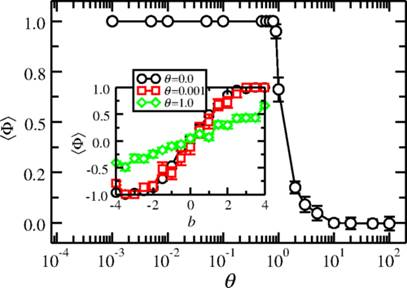FIG. 5.Average order parameter as a function of θ, for ρ=0.6, b=8, vd=6, and H=2. The inset shows the crossover from Φ=−1 to Φ=1 as b changes from −8 to 8, for different values of θ.